##### Trigonometry Workbook For DummiesWith the trigonometric substitution method, you can do integrals containing radicals of the following forms (given a is a constant and u is an expression containing x):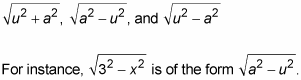You’re going to love this technique … about as much as sticking a hot poker in your eye.

Before you look at how trigonometric substitution works, here are some mnemonic tricks to help you keep this method straight. Remember, with mnemonic devices, silly (and vulgar) works. First, this involves three trig functions, tangent, sine, and secant. Their initial letters, t, s, and s, are the same letters as the initial letters of the name of this technique, trigonometric substitution. Pretty nice, eh?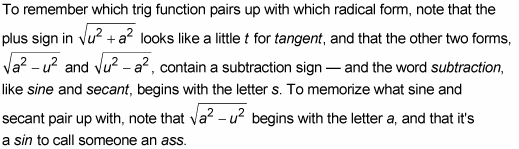Okay, this sin/ass mnemonic is admittedly pretty weak. If you can come up with a better mnemonic, use it!

Now, ready to do a problem?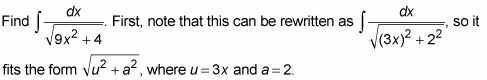1. Draw a right triangle—basically a SohCahToa triangle—where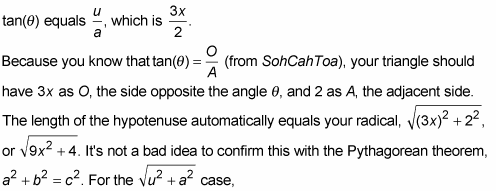a SohCahToa triangle is shown in the following figure.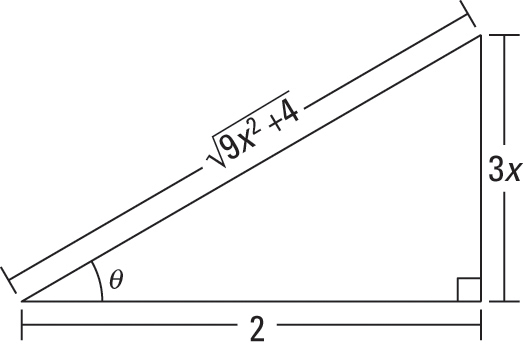2. Solve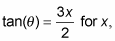then differentiate, and solve for dx.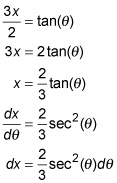3. Find which trig function is represented by the radical over the a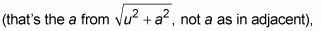and then solve for the radical.

Look at the triangle in the figure. The radical is the hypotenuse and a is 2, the adjacent side, so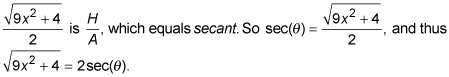4. Use the results from Steps 2 and 3 to make substitutions in the original problem and then integrate.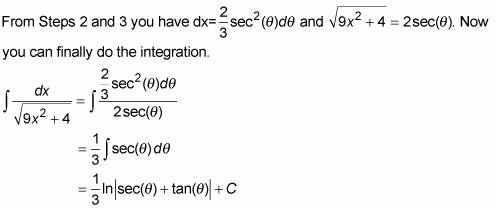5. Substitute the x expressions from Steps 1 and 3 back in for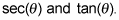You can also get the expressions from the triangle in the above figure.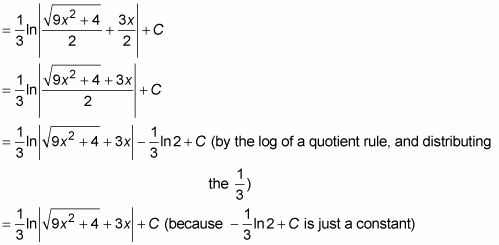Was that fun or what?